# usmap: Mapping the US

#### Extending plot_usmap with ggplot2

The nice thing about usmap::plot_usmap is it returns a ggplot object object, which means we can add ggplot layers to the plot right out of the box.

library(usmap)
library(ggplot2)

plot_usmap(regions = "counties") +
labs(title = "US Counties", subtitle = "This is a blank map of the counties of the United States.") +
theme(panel.background = element_rect(colour = "black", fill = "lightblue"))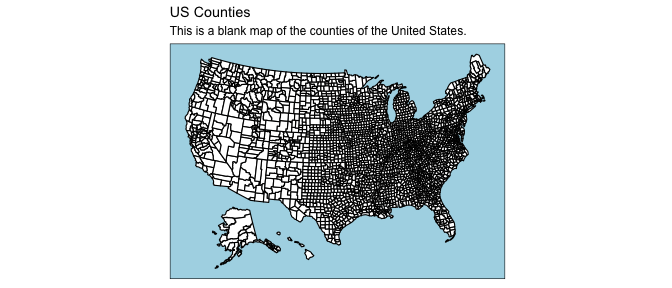#### Plot only certain states

library(usmap)
library(ggplot2)

plot_usmap(include = c("CA", "ID", "NV", "OR", "WA")) +
labs(title = "Western US States", subtitle = "These are the states in the Pacific Timezone.")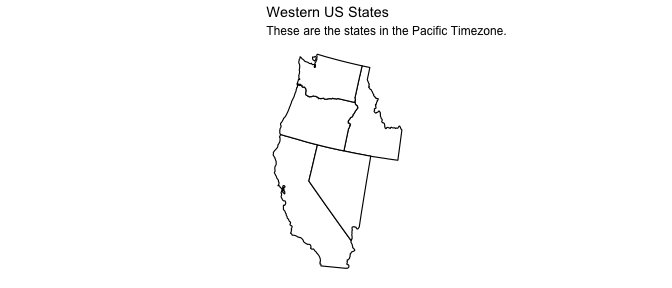#### Add some data to the map

library(usmap)
library(ggplot2)

plot_usmap(data = statepop, values = "pop_2015", lines = "red") +
scale_fill_continuous(name = "Population (2015)", label = scales::comma) +
theme(legend.position = "right")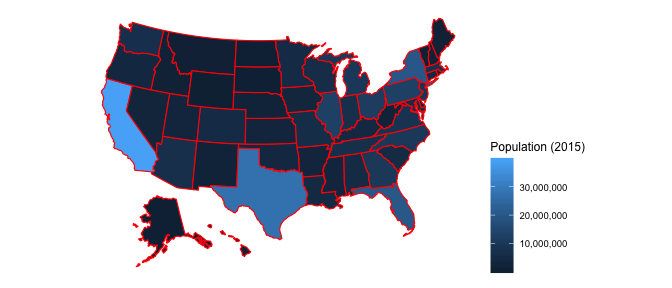Notice the comprehensive expandability that can be applied to the map using ggplot2 layers. For example, we might want to use a different color scheme.

#### Change fill color scale

library(usmap)
library(ggplot2)

plot_usmap(data = statepop, values = "pop_2015", lines = "red") +
scale_fill_continuous(
low = "white", high = "red", name = "Population (2015)", label = scales::comma
) + theme(legend.position = "right")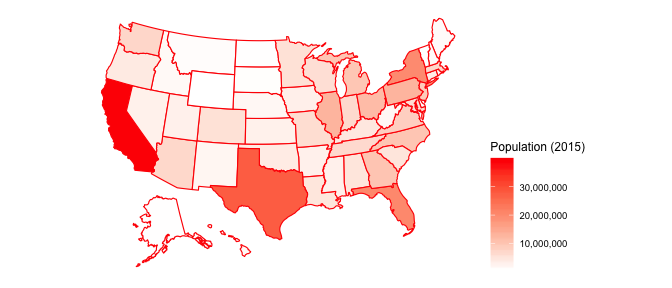The data-filled map can also be filtered to show certain regions only, like the western states shown above.

#### Show data in certain states

library(usmap)
library(ggplot2)

plot_usmap(
data = statepop, values = "pop_2015", include = c("CA", "ID", "NV", "OR", "WA"), lines = "red"
) +
scale_fill_continuous(
low = "white", high = "red", name = "Population (2015)", label = scales::comma
) +
labs(title = "Western US States", subtitle = "These are the states in the Pacific Timezone.") +
theme(legend.position = "right")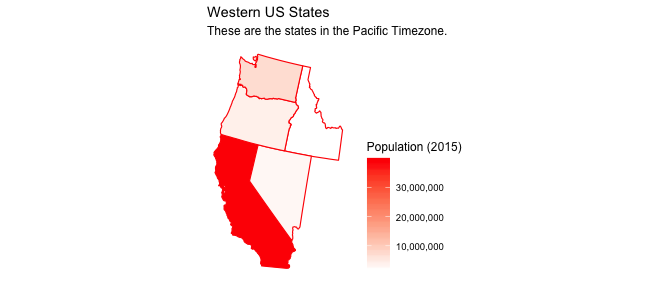## Raw map data

The raw US map data for counties or states can be obtained for further manipulation (and joining with data).

str(usmap::us_map())
#> 'data.frame':    12999 obs. of  9 variables:
#>  $long : num 1091779 1091268 1091140 1090940 1090913 ... #>$ lat  : num  -1380695 -1376372 -1362998 -1343517 -1341006 ...
#>  $order: int 1 2 3 4 5 6 7 8 9 10 ... #>$ hole : logi  FALSE FALSE FALSE FALSE FALSE FALSE ...
#>  $piece: int 1 1 1 1 1 1 1 1 1 1 ... #>$ group: chr  "01.1" "01.1" "01.1" "01.1" ...
#>  $fips : chr "01" "01" "01" "01" ... #>$ abbr : chr  "AL" "AL" "AL" "AL" ...
#>  $full : chr "Alabama" "Alabama" "Alabama" "Alabama" ... str(usmap::us_map(regions = "counties")) #> 'data.frame': 54187 obs. of 10 variables: #>$ long  : num  1225889 1244873 1244129 1272010 1276797 ...
#>  $lat : num -1275020 -1272331 -1267515 -1262889 -1295514 ... #>$ order : int  1 2 3 4 5 6 7 8 9 10 ...
#>  $hole : logi FALSE FALSE FALSE FALSE FALSE FALSE ... #>$ piece : int  1 1 1 1 1 1 1 1 1 1 ...
#>  $group : chr "01001.1" "01001.1" "01001.1" "01001.1" ... #>$ fips  : chr  "01001" "01001" "01001" "01001" ...
#>  $abbr : chr "AL" "AL" "AL" "AL" ... #>$ full  : chr  "Alabama" "Alabama" "Alabama" "Alabama" ...
#>  \$ county: chr  "Autauga County" "Autauga County" "Autauga County" "Autauga County" ...

You can also include only certain states and counties just like in plot_usmap. In fact, the regions and include parameters of plot_usmap are derived directly from their usage in us_map.